CSC 378: Data Structures and Algorithm Analysis

Leftist Trees

[L&D pg 304-307, Weiss 6.6]

# Mergeable Heaps

A "mergeable heap" is an ADT that stores an element from an ordered set, and supports the operations Insert, Min, and Extract-Min (just like priority queues). Mergeable heaps support an additional operation: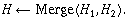Merge() takes two mergeable heaps and returns one that contains all of the elements. Duplicates are preserved.

Two implementations of the mergeable heap are the "leftist tree" and the "binomial heap". We'll talk about leftist trees in this lecture.

# Leftist Tree Definition

A "leftist tree" is an implementation of a mergeable heap.

In a binary tree, define an external node to be one with fewer than two children.

Define dist( i ) to be the number of edges on the shortest path from node i to a descendant external node.

A leftist tree is a binary tree with properties

1. key( i )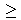key( parent( i ) )

The root contains the minimum key. As with array heaps, we could have the maximum at the top, simply by changing this property and modifying the code accordingly.
2. dist( right( i ) )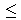dist( left( i ) )

The shortest path to a descendant external node is through the right child.

Here's a leftist tree: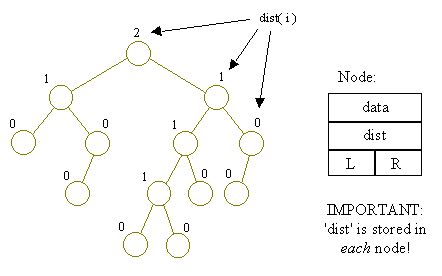Note that

• Every subtree is also a leftist tree, and
• dist( i ) = 1 + dist( right( i ) )

# Leftist Tree Operations

The operations supported by a leftist tree are: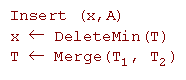Suppose that we already implemented the Merge procedure (below, we'll discuss the implementation). Merge can be used to make very simple Insert and DeleteMin procedures: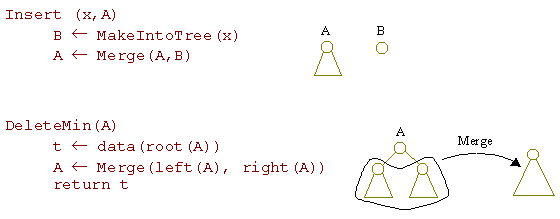## Merging Leftist Trees

The Merge procedure takes two leftist trees, A and B, and returns a leftist tree that contains the union of the elements of A and B. In a program, a leftist tree is represented by a pointer to its root.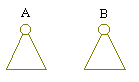In merging, the simplest case occurs when one tree is empty (i.e. the pointer to the root is NULL). In this case, just return the other.

Otherwise, what is the root of the merged tree?

It's A's root if A has the smaller key; it's B's root if B has the smaller key.

Suppose A's root has the smaller key. (If this isn't the case, simply swap the A and B pointers.) Then we can merge right(A) with B: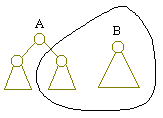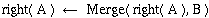Now right(A) has changed. Its dist() may have grown larger in the process, violating the second property above. If so, just swap right(A) and left(A):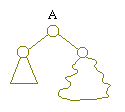if dist(right(A)) > dist(left(A)), swap right(A) and left(A).

Finally, since dist(right(A)) might have changed, we have to update dist(A):

dist(A)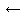1 + dist(right(A))

Of course, if right(A) = NULL, we'll assume that dist(right(A)) = -1.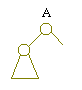dist(right(A)) = -1

So the whole Merge procedure looks like this: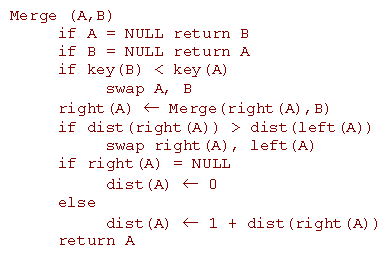Note: If a node's child is NULL, that child's dist is assumed to be -1. This isn't always checked in the code above!

## Analysis of Merge

Since each recursive call goes down either A's right path,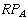, or B's right path,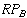, the number of calls is at most the sum of the lengths of the right paths: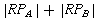and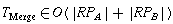since other operations in the body of Merge are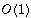. In other words,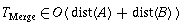,

since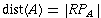. But what is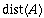in a tree of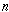nodes? Let's look at some examples:
 if dist(A) = 0,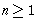.if dist(A) = 1,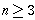.if dist(A) = 2,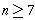.So the pattern is: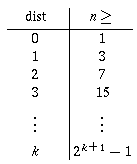So if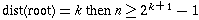. This makes sense, since the tree must be full down to the dist(A) level (otherwise, the second property would be violated!).

A full tree of dist(A) levels has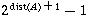nodes, so a general leftist tree ofnodes (which might have nodes dangling below the full levels) has: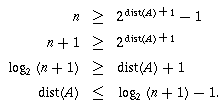Since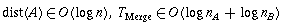. If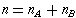, then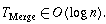As a consequence, the running times of Insert and DeleteMin are also in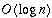.

## `Merge' in Action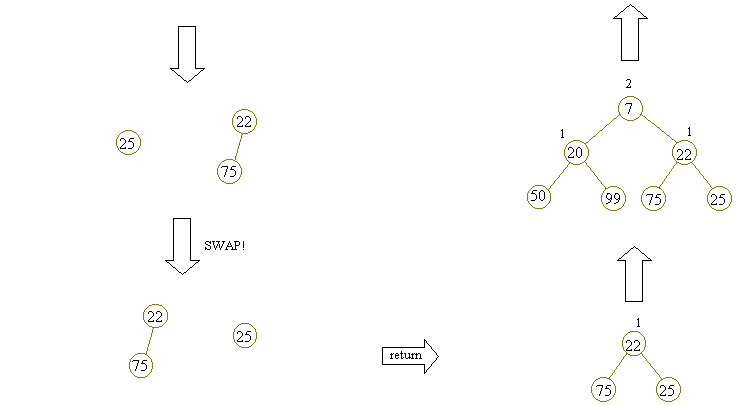Back to the lecture index